17 Nisan 2012 Salı

& the " W R i T E R i N A R T worK.. " ..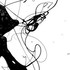murat danis
....muratdanis..THE ARTist  -PAiNTER-  ,  &  the  " W R i T E R   i  N     A R T   worK.. "  ..

1-..as a n  INDiViDUAL;  I have  M Y;     S H O W   C A S E;    T    E    A    M.....
2-....m y   show  g o e s ; UNDER ATOMiC  W O R L Ds .. (while ARTSLANT T E A M' s  " SHOW CASE "  trawel to the SKy..)
3-....m y   " C A S E  "   has  a  intension;     T O;  c r e a t e   a  " HOLy  GRAiL "    ;O U T   o f;    a   normal  World ART' s;  " CASE "..
...an EXAMPLE; to  m  y;  iNDiViVUAL;  S H O W    C A S E..

..the  ORiGiNAL  " C A S E ";  (  the Original ART worK..)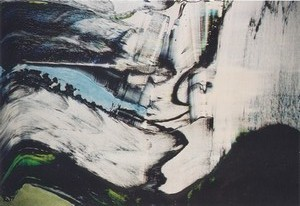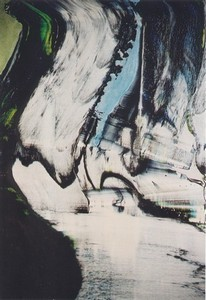t h   e   n;   " W R i T i N G;  i  n    this  ART WORK;"   ( the  C A S E )   b e g i   n  s..

..t h e  N A M E;( the Subject  -the TOPiC- )         o f  the        " S H O W    C A S E " ;
..."t h e  C O N Q U E R;   o    f    the   i S T A N B U L.."   (  "SLEEPERs i n  T H E   S N O W " )..
...there  w e r e   351,  WORKs;   E  n  t  e r   e  d;    the   showcase;    and   58 has passed;  t h e   first  STEP..
..t h e s e   a   r   e;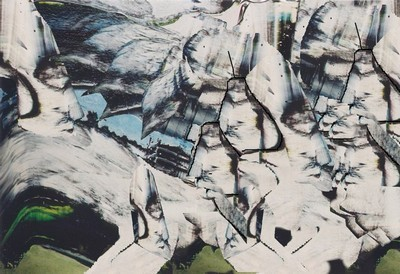the MATCH M A K E R;  B i r D..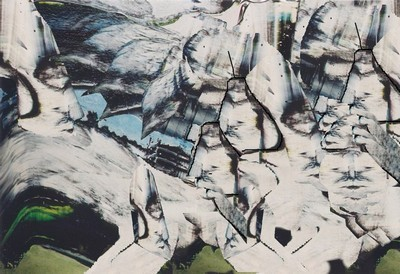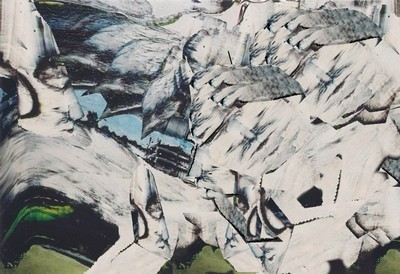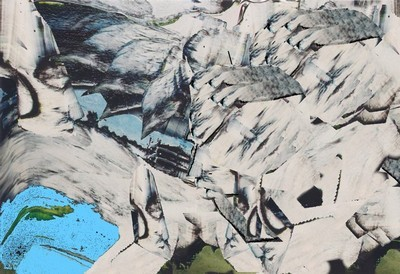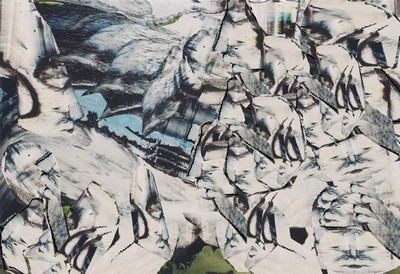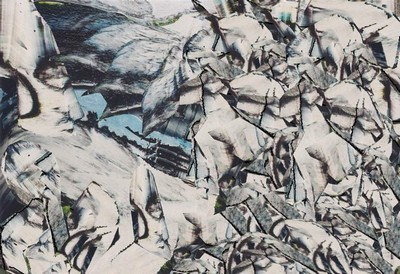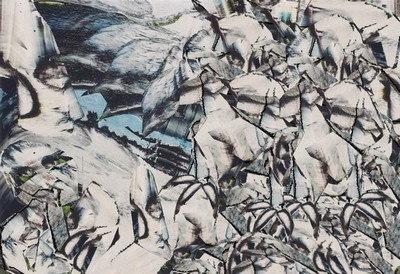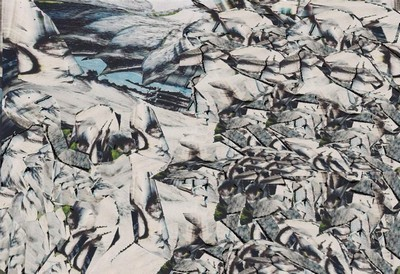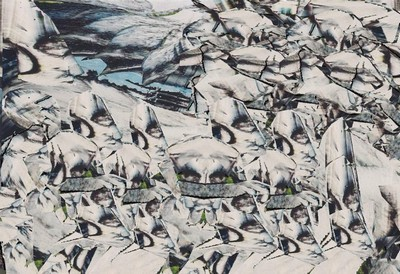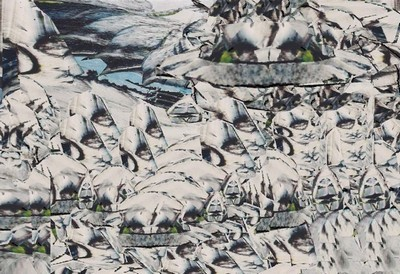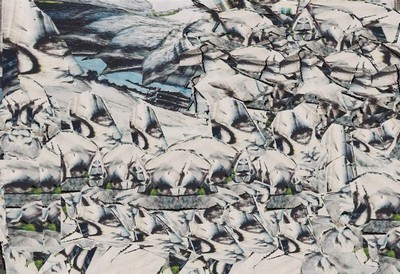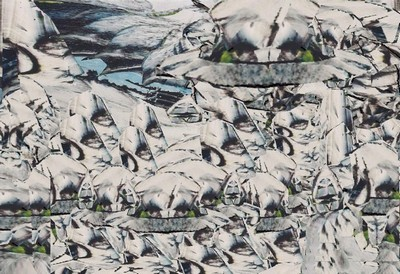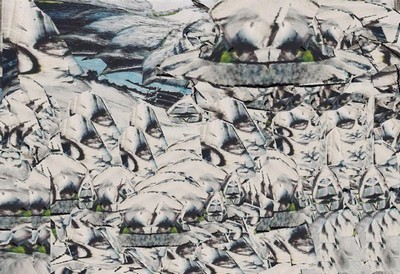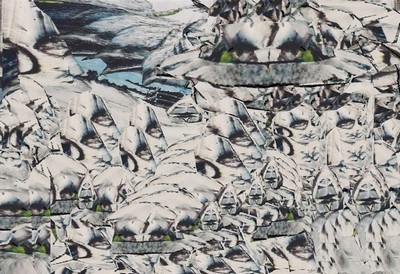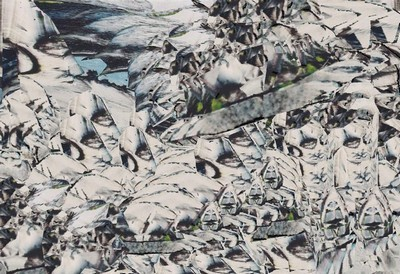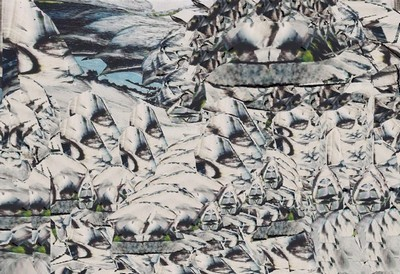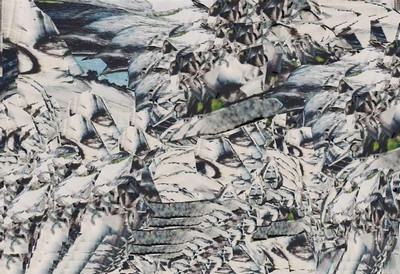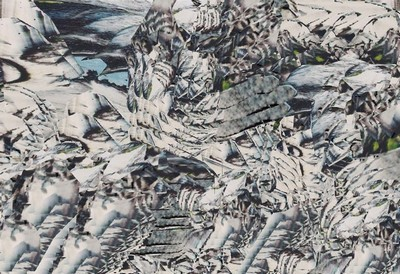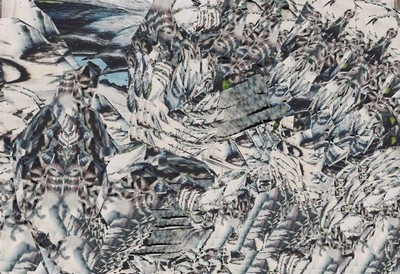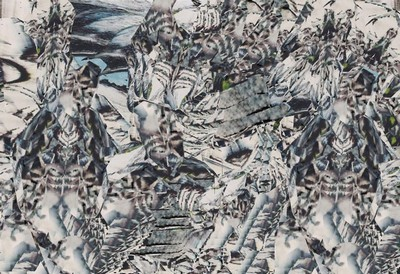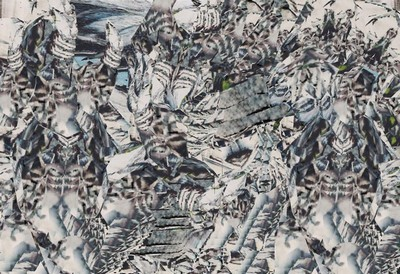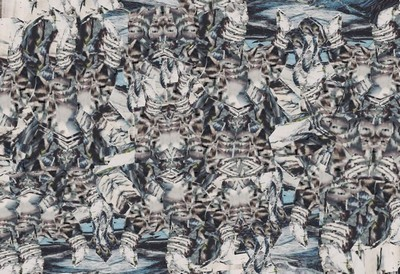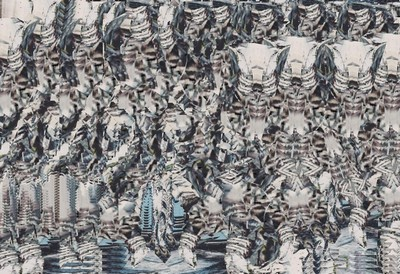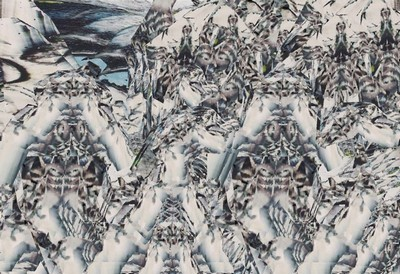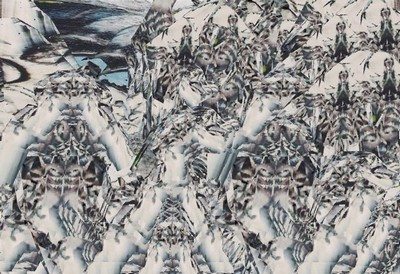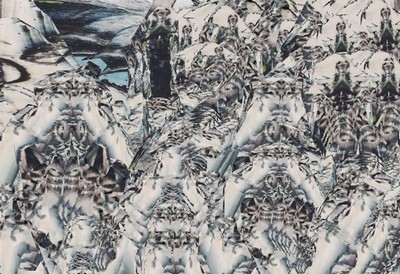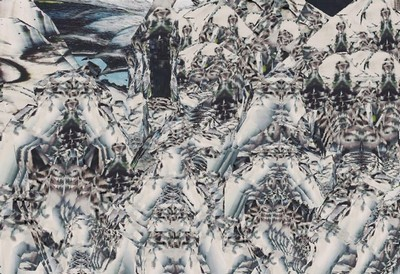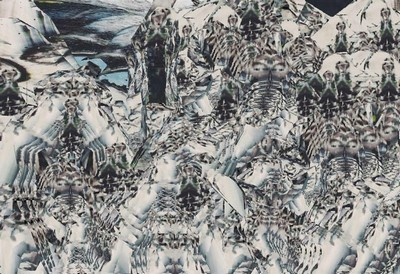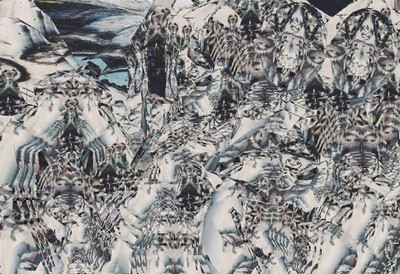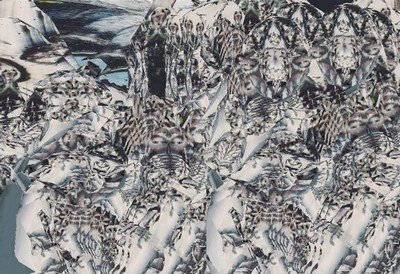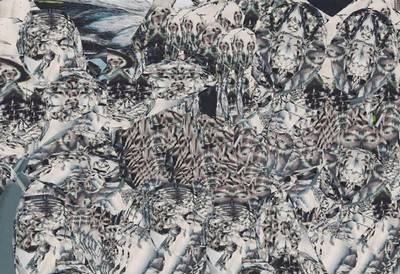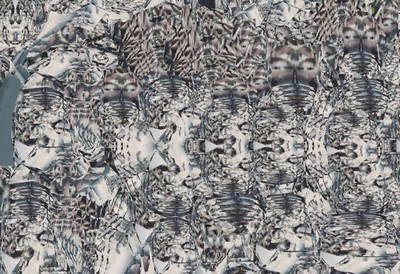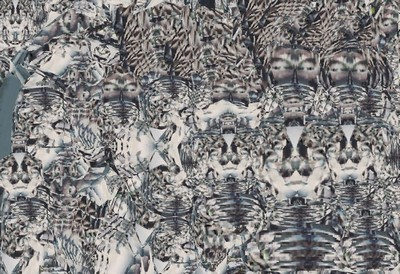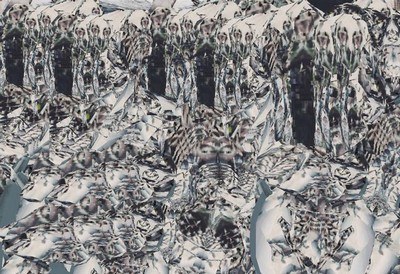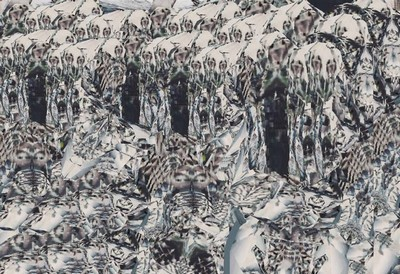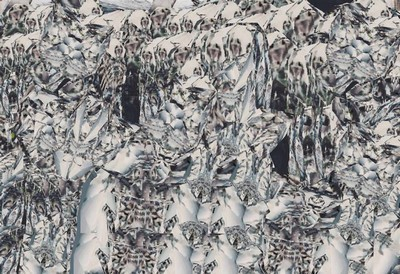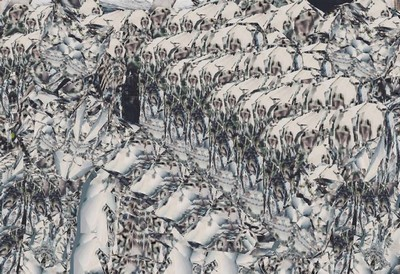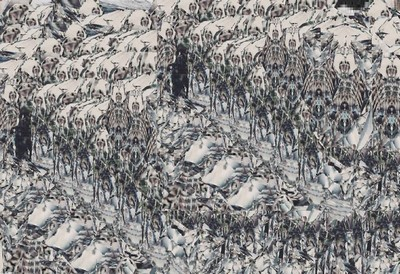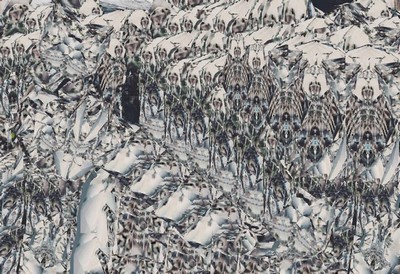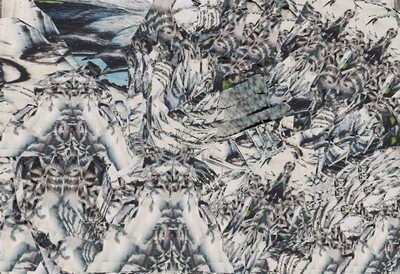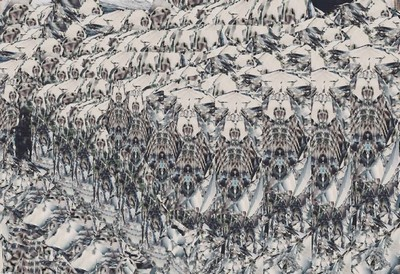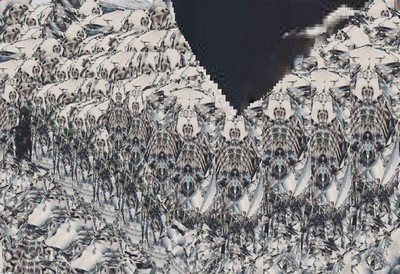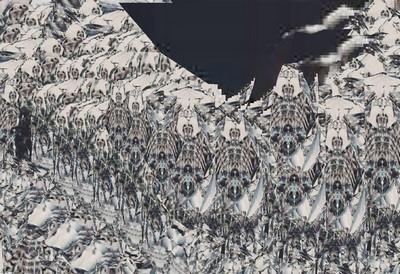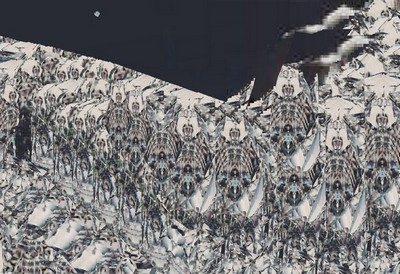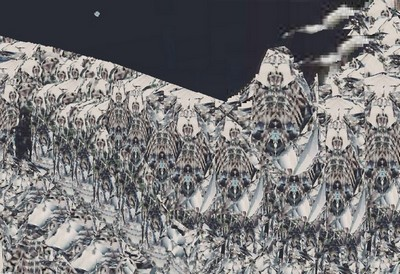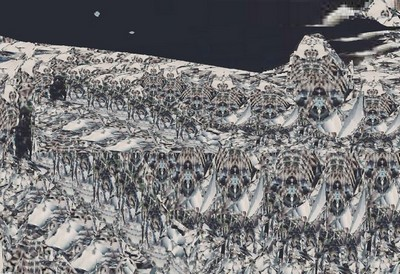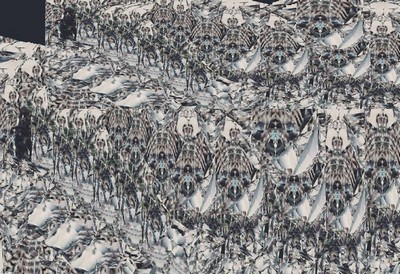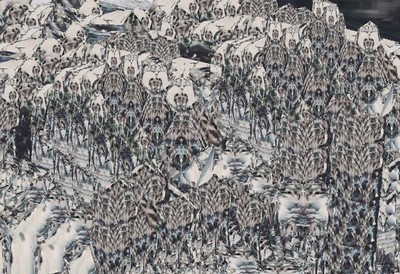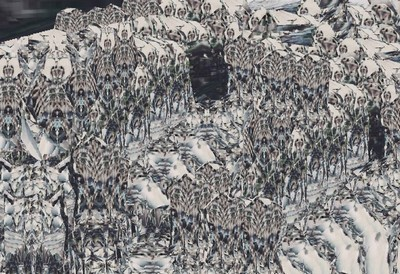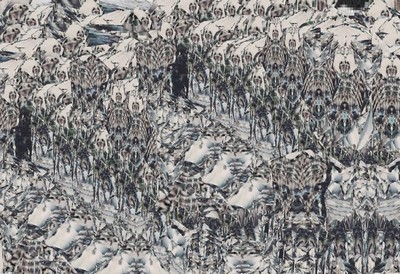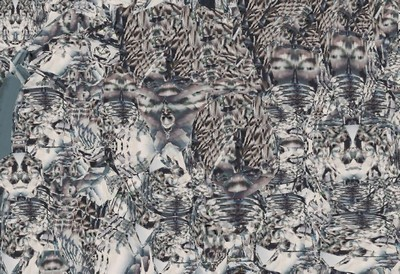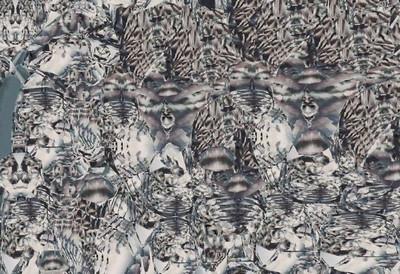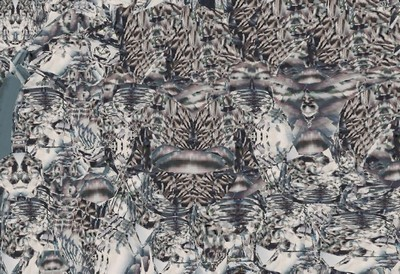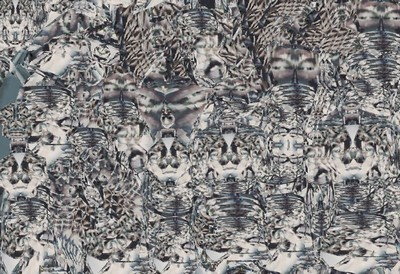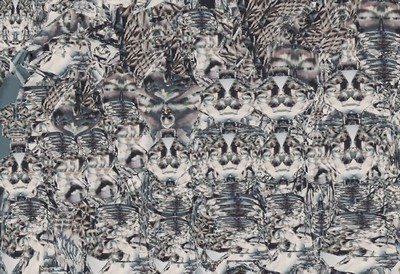.....this  w  a  s,  " m  y ;  STYLE ";       show  C A S E.
MY  OPiNiON;  ARTSLANT;  m u s t;   SELECT;  ONE ORiGiNAL;  ART WORK..with a prize..
...t h e n, the SECOND  R O U N D;        shall  b e g i n..
"  the M A S T E R   o f  A R T iSts,, SHOW C A S E; of         "HOLy    G R A L E..   -works-.. "
.....( this  selections  a b o v e;   -58 works-   has been taken  f r o m;  this " HOLY G R A L E ";      s e c t i O  N....)

S  O;  M A S T E R  of   A R T;  " S T A G E "   ;     n e e d S;
ARTiSTs;  t h a t  can  " W R i T E  in;     ARTwork..